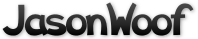Got questions, comments, patches, etc.? Contact Jason Woofenden
diff --git a/main.c b/main.c
index e3a0f83..91af524 100644 (file)
--- a/main.c
+++ b/main.c
#include <stdlib.h>
#include <string.h>

-#include "SFont.h"
+#include "font.h"

#include "args.h"
#include "common.h"
#include "config.h"
#include "dust.h"
#include "file.h"
+#include "float.h"
#include "globals.h"
#include "mt.h"
#include "rocks.h"
#include "score.h"
#include "sprite.h"
#include "sound.h"
-
-#ifdef WIN32
-#define SDL_SetAlpha(surf, flag, alpha)
-#endif
+#include "autopilot.h"

// ************************************* VARS
// SDL_Surface global variables
@@ -59,32 +57,40 @@ SDL_Surface
*surf_font_big; // The big font

-SFont_Font *g_font;
+font *g_font;
+
+struct dot {
+       int active;
+       float x, y;
+       float dx, dy;
+       float mass;   // in DOT_MASS_UNITs
+       float decay;  // rate at which to reduce mass.
+       int heat;     // heat multiplier (color).
+};

-// Structure global variables
-struct enginedots edot[MAXENGINEDOTS], *dotptr = edot;
-struct bangdots bdot[MAXBANGDOTS], *bdotptr = bdot;
+struct dot edot[MAXENGINEDOTS], *dotptr = edot;
+struct dot bdot[MAXBANGDOTS];

// Other global variables
char topline;
char *initerror = "";

-struct ship ship = { SHIP, 0, NULL, XSIZE/2, YSIZE/2, SCREENDXMIN, 0.0 };
+struct ship ship = { SHIP, 0, NULL, XSIZE/2, YSIZE/2, BARRIER_SPEED, 0.0 };

-float screendx = SCREENDXMIN, screendy = 0.0;
-float back_dist;
+float screendx = BARRIER_SPEED, screendy = 0.0;

// all movement is based on t_frame.
+unsigned long frames, start, end;
float t_frame;  // length of this frame (in ticks = 1/20th second)  adjusted for gamespeed
int ms_frame;   // length of this frame (milliseconds)
int ms_end;     // end of this frame (milliseconds)

-int bang = false;
-float bangx, bangy, bangdx, bangdy;
+float gamespeed = 1.00;

int score;

int pausedown = 0, paused = 0;

@@ -123,7 +129,7 @@ char *data_dir;
extern char *optarg;
extern int optind, opterr, optopt;

-#define TO_TICKS(seconds) ((seconds)*20*opt_gamespeed)
+#define TO_TICKS(seconds) ((seconds)*20*gamespeed)

// ************************************* FUNCS

@@ -135,38 +141,114 @@ init_engine_dots() {
}
}

+
void
-new_bang_dots(int xbang, int ybang, int dx, int dy, SDL_Surface *s)
+new_engine_dots(void) {
+       int dir, i;
+       int n = t_frame * ENGINE_DOTS_PER_TIC;
+       float a, r;  // angle, random length
+       float dx, dy;
+       float hx, hy; // half ship width/height.
+       static const int s = { 2, 1, 0, 1 };
+       float time;
+       float accelh, accelv, past_ship_dx, past_ship_dy;
+
+       hx = ship.image->w / 2;
+       hy = ship.image->h / 2;
+
+       for(dir=0; dir<4; dir++) {
+               if(!(ship.jets & 1<<dir)) continue;
+
+               for(i = 0; i<n; i++) {
+                       if(dotptr->active == 0) {
+                               a = frnd()*M_PI + (dir-1)*M_PI_2;
+                               r = sin(frnd()*M_PI);
+                               dx = r * cos(a);
+                               dy = r * -sin(a);  // screen y is "backwards".
+
+                               dotptr->decay = 3;
+                               dotptr->heat = 6;
+
+                               // dot was created at a random time during the time span
+                               time = frnd() * t_frame; // this is how long ago
+
+                               // calculate how fast the ship was going when this engine dot was
+                               // created (as if it had a smooth acceleration). This is used in
+                               // determining the velocity of the dots, but not their starting
+                               // location.
+                               accelh = ((ship.jets >> 2) & 1) - (ship.jets & 1);
+                               accelh *= THRUSTER_STRENGTH * time;
+                               past_ship_dx = ship.dx - accelh;
+                               accelv = ((ship.jets >> 1) & 1) - ((ship.jets >> 3) & 1);
+                               accelv *= THRUSTER_STRENGTH * time;
+                               past_ship_dy = ship.dy - accelv;
+
+                               // the starting position (not speed) of the dot is calculated as
+                               // though the ship were traveling at a constant speed for this
+                               // t_frame.
+                               dotptr->x = (ship.x - (ship.dx - screendx) * time) + s[dir]*hx;
+                               dotptr->y = (ship.y - (ship.dy - screendy) * time) + s[(dir+1)&3]*hy;
+                               if(dir&1) {
+                                       dotptr->dx = past_ship_dx + 2*dx;
+                                       dotptr->dy = past_ship_dy + 20*dy;
+                                       dotptr->mass = 60 * fabs(dy);
+                               } else {
+                                       dotptr->dx = past_ship_dx + 20*dx;
+                                       dotptr->dy = past_ship_dy + 2*dy;
+                                       dotptr->mass = 60 * fabs(dx);
+                               }
+
+                               // move the dot as though it were created in the past
+                               dotptr->x += (dotptr->dx - screendx) * time;
+                               dotptr->y += (dotptr->dy - screendy) * time;
+
+                               if(!fclip(dotptr->x, XSIZE) && !fclip(dotptr->y, YSIZE)) {
+                                       dotptr->active = 1;
+                                       if(dotptr - edot < MAXENGINEDOTS-1) {
+                                               dotptr++;
+                                       } else {
+                                               dotptr = edot;
+                                       }
+                               }
+                       }
+               }
+       }
+}
+
+
+void
+new_bang_dots(struct sprite *s)
{
int i, n, x, y;
uint16_t *pixel, c;
uint32_t colorkey;
int row_inc;
double theta, r;
+       SDL_Surface *img = s->image;

-       n = 24.0 * t_frame;
-       pixel = s->pixels;
-       row_inc = s->pitch/sizeof(uint16_t) - s->w;
-       colorkey = s->format->colorkey;
+       n = 20;
+       pixel = img->pixels;
+       row_inc = img->pitch/sizeof(uint16_t) - img->w;
+       colorkey = img->format->colorkey;

-       SDL_LockSurface(s);
+       if(SDL_MUSTLOCK(img)) { SDL_LockSurface(img); }

for(i=0; i<n; i++) {
-               pixel = s->pixels;
-               for(y=0; y<s->h; y++) {
-                       for(x = 0; x<s->w; x++) {
+               pixel = img->pixels;
+               for(y=0; y<img->h; y++) {
+                       for(x = 0; x<img->w; x++) {
c = *pixel++;
if(c && c != colorkey) {
theta = frnd()*M_PI*2;
r = frnd(); r = 1 - r*r;

-                                       bdot[bd2].dx = 45*r*cos(theta) + dx;
-                                       bdot[bd2].dy = 45*r*sin(theta) + dy;
-                                       bdot[bd2].x = x + xbang;
-                                       bdot[bd2].y = y + ybang;
-                                       bdot[bd2].c = (i < n-3) ? 0 : c;
-                                       bdot[bd2].life = 100;
-                                       bdot[bd2].decay = frnd()*3 + 1;
+                                       bdot[bd2].dx = 45*r*cos(theta) + s->dx;
+                                       bdot[bd2].dy = 45*r*sin(theta) + s->dy;
+                                       bdot[bd2].x = x + s->x;
+                                       bdot[bd2].y = y + s->y;
+                                       bdot[bd2].mass = frnd() * 99;
+                                       bdot[bd2].decay = frnd()*1.5 + 0.5;
+                                       bdot[bd2].heat = 3;
bdot[bd2].active = 1;

bd2 = (bd2+1) % MAXBANGDOTS;
@@ -176,180 +258,67 @@ new_bang_dots(int xbang, int ybang, int dx, int dy, SDL_Surface *s)
}
}

-       SDL_UnlockSurface(s);
+       if(SDL_MUSTLOCK(img)) { SDL_UnlockSurface(img); }
}

+
void
-draw_bang_dots(SDL_Surface *s)
+move_dot(struct dot *d)
{
-       int i;
-       int first_i, last_i;
-       uint16_t *pixels, *pixel, c;
-       int row_inc = s->pitch/sizeof(uint16_t);
Sprite *hit;
-
-       pixels = (uint16_t *) s->pixels;
-       first_i = -1;
-       last_i = 0;
-
-       for(i=0; i<MAXBANGDOTS; i++) {
-               if(!bdot[i].active) continue;
-
-               // decrement life and maybe kill
-               bdot[i].life -= bdot[i].decay;
-               if(bdot[i].life<0) { bdot[i].active = 0; continue; }
-
-               // move and clip
-               bdot[i].x += (bdot[i].dx - screendx)*t_frame;
-               bdot[i].y += (bdot[i].dy - screendy)*t_frame;
-               if(bdot[i].x < 0 || bdot[i].x >= XSIZE || bdot[i].y < 0 || bdot[i].y >= YSIZE) {
-                       bdot[i].active = 0;
-                       continue;
-               }
-
-               // check collisions
-               if((hit = pixel_collides(bdot[i].x, bdot[i].y))) {
-                       if(hit->type != SHIP) { // they shouldn't hit the ship, but they do
-                               bdot[i].active = 0;
-                               hit->dx += ENGINE_DOT_WEIGHT * bdot[i].life * bdot[i].dx / sprite_mass(hit);
-                               hit->dy += ENGINE_DOT_WEIGHT * bdot[i].life * bdot[i].dy / sprite_mass(hit);
-                               continue;
+       float mass;
+
+       if(d->active) {
+               d->x += (d->dx - screendx) * t_frame;
+               d->y += (d->dy - screendy) * t_frame;
+               d->mass -= t_frame * d->decay;
+               if(d->mass < 0 || fclip(d->x, XSIZE) || fclip(d->y, YSIZE))
+                       d->active = 0;
+               else {
+                       hit = pixel_collides(d->x, d->y);
+                       if(hit) if(hit->type != SHIP) {
+                               d->active = 0;
+                               mass = sprite_mass(hit);
+                               hit->dx += DOT_MASS_UNIT * d->mass * (d->dx - hit->dx) / mass;
+                               hit->dy += DOT_MASS_UNIT * d->mass * (d->dy - hit->dy) / mass;
}
}
-
-               pixel = pixels + row_inc*(int)(bdot[i].y) + (int)(bdot[i].x);
-               if(bdot[i].c) c = bdot[i].c; else c = heatcolor[(int)(bdot[i].life)*3];
-               *pixel = c;
}
}

-
void
-new_engine_dots(float time_span, int dir) {
+move_dots(void)
+{
int i;
-       int n = time_span * ENGINE_DOTS_PER_TIC;
-       float a, r;  // angle, random length
-       float dx, dy;
-       float hx, hy; // half ship width/height.
-       static const int s = { 2, 1, 0, 1 };
-       float time;
-       float accelh, accelv, past_ship_dx, past_ship_dy;
-
-       hx = ship.image->w / 2;
-       hy = ship.image->h / 2;

-       for(i = 0; i<n; i++) {
-               if(dotptr->active == 0) {
-                       a = frnd()*M_PI + (dir-1)*M_PI_2;
-                       r = sin(frnd()*M_PI);
-                       dx = r * cos(a);
-                       dy = r * -sin(a);  // screen y is "backwards".
-
-                       dotptr->active = 1;
-
-                       // dot was created at a random time during the time span
-                       time = frnd() * time_span; // this is how long ago
-
-                       // calculate how fast the ship was going when this engine dot was
-                       // created (as if it had a smoothe accelleration). This is used in
-                       // determining the velocity of the dots, but not their starting
-                       // location.
-                       accelh = ((ship.jets >> 2) & 1) - (ship.jets & 1);
-                       accelh *= THRUSTER_STRENGTH * time;
-                       past_ship_dx = ship.dx - accelh;
-                       accelv = ((ship.jets >> 1) & 1) - ((ship.jets >> 3) & 1);
-                       accelv *= THRUSTER_STRENGTH * time;
-                       past_ship_dy = ship.dy - accelv;
-
-                       // the starting position (not speed) of the dot is calculated as
-                       // though the ship were traveling at a constant speed for this
-                       // time_span.
-                       dotptr->x = (ship.x - (ship.dx - screendx) * time) + s[dir]*hx;
-                       dotptr->y = (ship.y - (ship.dy - screendy) * time) + s[(dir+1)&3]*hy;
-                       if(dir&1) {
-                               dotptr->dx = past_ship_dx + 2*dx;
-                               dotptr->dy = past_ship_dy + 20*dy;
-                               dotptr->life = 60 * fabs(dy);
-                       } else {
-                               dotptr->dx = past_ship_dx + 20*dx;
-                               dotptr->dy = past_ship_dy + 2*dy;
-                               dotptr->life = 60 * fabs(dx);
-                       }
-
-                       // move the dot as though it were created in the past
-                       dotptr->x += (dotptr->dx - screendx) * time;
-                       dotptr->y += (dotptr->dy - screendy) * time;
-
-                       if(dotptr - edot < MAXENGINEDOTS-1) dotptr++;
-                       else dotptr = edot;
-               }
-       }
+       for(i=0; i<MAXBANGDOTS; i++) move_dot(&bdot[i]);
+       for(i=0; i<MAXENGINEDOTS; i++) move_dot(&edot[i]);
}

-void
-move_engine_dots() {
-       int i;
-       for(i = 0; i<MAXENGINEDOTS; i++) {
-               if(!edot[i].active) {
-                       continue;
-               }
-               edot[i].x += (edot[i].dx - screendx)*t_frame;
-               edot[i].y += (edot[i].dy - screendy)*t_frame;
-               edot[i].life -= t_frame*3;
-               if(edot[i].life < 0 || edot[i].x<0 || edot[i].x >= XSIZE || edot[i].y < 0 || edot[i].y >= YSIZE) {
-                       edot[i].active = 0;
-               }
-       }
-}

void
-draw_engine_dots(SDL_Surface *s) {
-       int i;
-       uint16_t c;
-       uint16_t *pixels = (uint16_t *) s->pixels;
-       int row_inc = s->pitch/sizeof(uint16_t);
-       int heatindex;
-       Sprite *hit;
+draw_dot(struct dot *d)
+{
+       uint16_t *pixels, *pixel;
+       int row_inc;

-       for(i = 0; i<MAXENGINEDOTS; i++) {
-               if(!edot[i].active) {
-                       continue;
-               }
-               // check collisions
-               if((hit = pixel_collides(edot[i].x, edot[i].y))) {
-                       if(hit->type != SHIP) { // they shouldn't hit the ship, but they do
-                               edot[i].active = 0;
-                               hit->dx += ENGINE_DOT_WEIGHT * edot[i].life * edot[i].dx / sprite_mass(hit);
-                               hit->dy += ENGINE_DOT_WEIGHT * edot[i].life * edot[i].dy / sprite_mass(hit);
-                               continue;
-                       }
-               }
-               heatindex = edot[i].life * 6;
-               c = heatindex>3*W ? heatcolor[3*W-1] : heatcolor[heatindex];
-               pixels[row_inc*(int)(edot[i].y) + (int)(edot[i].x)] = c;
+       if(d->active) {
+               pixels = (uint16_t *) surf_screen->pixels;
+               row_inc = surf_screen->pitch / sizeof(uint16_t);
+               pixel = pixels + (int)d->y * row_inc + (int)d->x;
+               *pixel = heatcolor[min(3*W-1, (int)(d->mass * d->heat))];
}
}

void
-drawdots(SDL_Surface *s) {
-       int m;
-
-       move_engine_dots();
-
-       // Create engine dots
-       for(m = 0; m<4; m++) {
-               if(ship.jets & 1<<m) { // 'jets' is a bit field
-                       new_engine_dots(t_frame,m);
-               }
-       }
-
-       move_dust();
+draw_dots(void) {
+       int i;

-       SDL_LockSurface(s);
-       draw_dust(s);
-       draw_engine_dots(s);
-       draw_bang_dots(s);
-       SDL_UnlockSurface(s);
+       if(SDL_MUSTLOCK(surf_screen)) { SDL_LockSurface(surf_screen); }
+       draw_dust();
+       for(i=0; i<MAXBANGDOTS; i++) draw_dot(&bdot[i]);
+       for(i=0; i<MAXENGINEDOTS; i++) draw_dot(&edot[i]);
+       if(SDL_MUSTLOCK(surf_screen)) { SDL_UnlockSurface(surf_screen); }
}

SDL_Surface *
}

+void font_cleanup() {
+       font_free(g_font);
+}
+
int
init(void) {

@@ -436,9 +409,11 @@ init(void) {
if(s) {
-               free(s);
-               g_font = SFont_InitFont(surf_font_big);
+               atexit(&font_cleanup);
+       } else {
+               fprintf(stderr, "could create path to font\n");
+               exit(1);
}

init_engine_dots();
@@ -474,22 +449,15 @@ draw_game_over(void)
int x;
char *text0, *text1;
SDL_Rect dest;
-       float a_game = 0, a_over = 0;
-
-       // fade in "GAME", then "OVER".

dest.x = (XSIZE-surf_b_game->w)/2;
dest.y = (YSIZE-surf_b_game->h)/2-40;
-       SDL_SetAlpha(surf_b_game, SDL_SRCALPHA, (int)(a_game*(200 + 55*cos(fadetimer))));
SDL_BlitSurface(surf_b_game,NULL,surf_screen,&dest);

dest.x = (XSIZE-surf_b_over->w)/2;
dest.y = (YSIZE-surf_b_over->h)/2 + 40;
-       SDL_SetAlpha(surf_b_over, SDL_SRCALPHA, (int)(a_over*(200 + 55*sin(fadetimer))));
SDL_BlitSurface(surf_b_over,NULL,surf_screen,&dest);

if(new_high_score(score)) {
@@ -500,11 +468,11 @@ draw_game_over(void)
text1 = msgs[g_easy];
}

-       x = (XSIZE-SFont_TextWidth(g_font,text0))/2 + cos(fadetimer/9)*10;
+       x = (XSIZE-font_width(text0))/2 + cos(fadetimer/9)*10;

-       x = (XSIZE-SFont_TextWidth(g_font,text1))/2 + sin(fadetimer/9)*10;
+       x = (XSIZE-font_width(text1))/2 + sin(fadetimer/9)*10;
}

void
@@ -518,80 +486,85 @@ draw_title_page(void)

-       SDL_SetAlpha(surf_b_variations, SDL_SRCALPHA, (int)(200 + 55*sin(fadetimer)));
SDL_BlitSurface(surf_b_variations,NULL,surf_screen,&dest);

dest.x = (XSIZE-surf_b_on->w)/2 + cos((fadetimer + 1.0)/6.5)*10;
dest.y = (YSIZE/2-surf_b_on->h)/2 + surf_b_variations->h + 20 + sin((fadetimer + 1.0)/5.0)*10;
-       SDL_SetAlpha(surf_b_on, SDL_SRCALPHA, (int)(200 + 55*sin(fadetimer-1.0)));
SDL_BlitSurface(surf_b_on,NULL,surf_screen,&dest);

dest.x = (XSIZE-surf_b_rockdodger->w)/2 + cos((fadetimer + 2.0)/6.5)*10;
dest.y = (YSIZE/2-surf_b_rockdodger->h)/2 + surf_b_variations->h + surf_b_on->h + 40 + sin((fadetimer + 2.0)/5)*10;
-       SDL_SetAlpha(surf_b_rockdodger, SDL_SRCALPHA, (int)(200 + 55*sin(fadetimer-2.0)));
SDL_BlitSurface(surf_b_rockdodger,NULL,surf_screen,&dest);

-       x = (XSIZE-SFont_TextWidth(g_font,text))/2 + cos(fadetimer/4.5)*10;
+       x = (XSIZE-font_width(text))/2 + cos(fadetimer/4.5)*10;

text = "Version " VERSION;
-       x = (XSIZE-SFont_TextWidth(g_font,text))/2 + sin(fadetimer/4.5)*10;
+       x = (XSIZE-font_width(text))/2 + sin(fadetimer/4.5)*10;
}

void
-draw(void) {
-
+draw(void)
+{
SDL_FillRect(surf_screen,NULL,0);  // black background
-       drawdots(surf_screen);             // background dots
+       draw_dots();            // background dots
draw_sprite(SPRITE(&ship));
draw_rocks();

show_lives();
show_score();

-       // If it's game over, show the game over graphic in the dead centre
switch (state) {
case GAME_OVER: draw_game_over(); break;

case TITLE_PAGE: draw_title_page(); break;

-               case HIGH_SCORE_ENTRY:
-                       play_tune(TUNE_HIGH_SCORE_ENTRY);
-               // FALL THROUGH TO
+               case HIGH_SCORE_ENTRY: play_tune(TUNE_HIGH_SCORE_ENTRY);
+                       // and fall through to
case HIGH_SCORE_DISPLAY:
// Display de list o high scores mon.
-                       display_scores(surf_screen, 150,50);
+                       display_scores(150,50);
break;
case GAMEPLAY:
; // no action necessary
}

-       collisions();
-
-       ms_frame = SDL_GetTicks() - ms_end;
-       ms_end += ms_frame;
-       t_frame = opt_gamespeed * ms_frame / 50;
-       if(state == GAMEPLAY) score += ms_frame;
-
// Update the surface
SDL_Flip(surf_screen);
}

static inline void
-kill_ship(Sprite *ship)
+kill_ship(struct ship *ship)
{
-       ship->flags = MOVE;
-       bang = true;
+       play_sound(SOUND_BANG);
+       new_bang_dots(SPRITE(ship));
+       if(--ship->lives) {
+               // want ship to be invisible, but keep drifting at sqrt(speed)
+               // to leave it in the middle of the space from the explosion.
+               ship->flags = MOVE;
+               ship->dx = (ship->dx < 0) ? -sqrt(-ship->dx) : sqrt(ship->dx);
+               ship->dy = (ship->dy < 0) ? -sqrt(-ship->dy) : sqrt(ship->dy);
+               if(ship->dx < BARRIER_SPEED) ship->dx = BARRIER_SPEED;
+       } else {
+               state = GAME_OVER;
+               state_timeout = 200.0;
+               ship->flags = 0;
+               // scrolling is based on the ship speed, so we need to reset it.
+               ship->dx = BARRIER_SPEED; ship->dy = 0;
+       }
}

void
do_collision(Sprite *a, Sprite *b)
{
-       if(a->type == SHIP) kill_ship(a);
-       else if (b->type == SHIP) kill_ship(b);
+       if(a->type == SHIP) kill_ship((struct ship *)a);
+       else if(b->type == SHIP) kill_ship((struct ship *)b);
else bounce(a, b);
}

@@ -607,24 +580,66 @@ init_score_entry(void)
insert_score(score);
}

+// Count down the state timer, and change state when it gets to zero or less;
+void
+update_state(void)
+{
+       state_timeout -= t_frame*3;
+       if(state_timeout > 0) return;
+
+       switch(state) {
+               case GAMEPLAY: break;  // no action necessary
+                       // Restore the ship and continue playing
+                       ship.flags = DRAW|MOVE|COLLIDE;
+                       state = GAMEPLAY;
+                       play_tune(TUNE_GAMEPLAY);
+                       break;
+               case GAME_OVER:
+                       if(new_high_score(score)) init_score_entry();
+                       else {
+                               state = HIGH_SCORE_DISPLAY;
+                               state_timeout = 400;
+                       }
+                       break;
+               case HIGH_SCORE_DISPLAY:
+                       state = TITLE_PAGE;
+                       state_timeout = 600.0;
+                       break;
+               case HIGH_SCORE_ENTRY:
+                       break;
+               case TITLE_PAGE:
+                       state = HIGH_SCORE_DISPLAY;
+                       state_timeout = 200.0;
+                       break;
+       }
+}
+
void
gameloop() {
SDL_Event e;
Uint8 *keystate;
float tmp;

-
for(;;) {
+               ms_frame = SDL_GetTicks() - ms_end;
+               ms_end += ms_frame;
+               if(ms_frame > 50) {
+                       ms_frame = 50;
+               }
+               t_frame = gamespeed * ms_frame / 50;
+               frames++;
+
+               if(opt_autopilot) {
+                       autopilot(t_frame);
+               }
+
while(SDL_PollEvent(&e)) {
switch(e.type) {
case SDL_QUIT: return;
-                               case SDL_KEYUP:
-                                       if(e.key.keysym.sym == SDLK_ESCAPE
-                                          || e.key.keysym.sym == SDLK_q)
-                                               return;
-                                       break;
case SDL_KEYDOWN:
-                                       if(state == HIGH_SCORE_ENTRY)
+                                       if(state == HIGH_SCORE_ENTRY) {
if(!process_score_input(&e.key.keysym)) {
// Write the high score table to the file
write_high_score_table();
@@ -633,105 +648,88 @@ gameloop() {
state_timeout = 200;
play_tune(TUNE_TITLE_PAGE);
}
+                                       } else if(e.key.keysym.sym == SDLK_q) {
+                                               return;
+                                       }
+
+                                       if(e.key.keysym.sym == SDLK_ESCAPE) {
+                                               return;
+                                       }
break;
}
}
keystate = SDL_GetKeyState(NULL);
+               if(opt_autopilot) {
+                       autopilot_fix_keystates(keystate);
+               }

-               if(!paused) {
-                       // Count down the game loop timer, and change state when it gets to zero or less;
-
-                       if((state_timeout -= t_frame*3) < 0) {
-                               switch(state) {
-                                               // Restore the ship and continue playing
-                                               ship.flags = DRAW|MOVE|COLLIDE;
-                                               state = GAMEPLAY;
-                                               play_tune(TUNE_GAMEPLAY);
-                                               break;
-                                       case GAME_OVER:
-                                               if(new_high_score(score)) init_score_entry();
-                                               else {
-                                                       state = HIGH_SCORE_DISPLAY;
-                                                       state_timeout = 400;
-                                               }
-                                               break;
-                                       case HIGH_SCORE_DISPLAY:
-                                               state = TITLE_PAGE;
-                                               state_timeout = 600.0;
-                                               break;
-                                       case HIGH_SCORE_ENTRY:
-                                               break;
-                                       case TITLE_PAGE:
-                                               state = HIGH_SCORE_DISPLAY;
-                                               state_timeout = 200.0;
-                                               break;
-                                       case GAMEPLAY:
-                                               ; // no action necessary
+               if(state == GAMEPLAY) {
+                       if(!paused) {
+                               score += ms_frame;
+
+                               if(keystate[SDLK_LEFT]  || keystate[SDLK_h]) { ship.dx -= THRUSTER_STRENGTH*t_frame; ship.jets |= 1<<0;}
+                               if(keystate[SDLK_DOWN]  || keystate[SDLK_t]) { ship.dy += THRUSTER_STRENGTH*t_frame; ship.jets |= 1<<1;}
+                               if(keystate[SDLK_RIGHT] || keystate[SDLK_n]) { ship.dx += THRUSTER_STRENGTH*t_frame; ship.jets |= 1<<2;}
+                               if(keystate[SDLK_UP]    || keystate[SDLK_c]) { ship.dy -= THRUSTER_STRENGTH*t_frame; ship.jets |= 1<<3;}
+                               if(ship.jets) {
+                                       ship.dx = fconstrain2(ship.dx, -50, 50);
+                                       ship.dy = fconstrain2(ship.dy, -50, 50);
}
-                       } else {
-                                       if(bangx < 60) bangx = 60;
+                               if(keystate[SDLK_3])            { SDL_SaveBMP(surf_screen, "snapshot.bmp"); }
+                       }
+
+                       if(keystate[SDLK_p] | keystate[SDLK_s]) {
+                               if(!pausedown) {
+                                       paused = !paused;
+                                       pausedown = 1;
+                                       if(!paused) ms_end = SDL_GetTicks();
}
+                       } else {
+                               pausedown = 0;
}
+               }

-                       new_rocks();
+               if(!paused) {
+                       update_state();

// SCROLLING
-                       tmp = (ship.y+ship.dy*t_frame-YSCROLLTO)/25 + (ship.dy-screendy);
+                       tmp = (ship.y+ship.h/2 + ship.dy*t_frame - YSCROLLTO)/25 + (ship.dy-screendy);
screendy += tmp * t_frame/12;
-                       tmp = (ship.x+ship.dx*t_frame-XSCROLLTO)/25 + (ship.dx-screendx);
+                       tmp = (ship.x+ship.w/2 + ship.dx*t_frame - XSCROLLTO)/25 + (ship.dx-screendx);
screendx += tmp * t_frame/12;
// taper off so we don't hit the barrier abruptly.
// (if we would hit in < 2 seconds, adjust to 2 seconds).
-                       if(back_dist + (screendx - SCREENDXMIN)*TO_TICKS(2) < 0)
-                               screendx = SCREENDXMIN - (back_dist/TO_TICKS(2));
-                       back_dist += (screendx - SCREENDXMIN)*t_frame;
-
-                       // move bang center
-                       bangx += (bangdx - screendx)*t_frame;
-                       bangy += (bangdy - screendy)*t_frame;
+                       if(dist_ahead + (screendx - BARRIER_SPEED)*TO_TICKS(2) < 0)
+                               screendx = BARRIER_SPEED - (dist_ahead/TO_TICKS(2));
+                       dist_ahead += (screendx - BARRIER_SPEED)*t_frame;

move_sprites();
+                       move_dots();
+                       move_dust();

+                       new_rocks();

// BOUNCE off left or right edge of screen
if(ship.x < 0 || ship.x+ship.w > XSIZE) {
ship.x -= (ship.dx-screendx)*t_frame;
-                               ship.dx = screendx - (ship.dx-screendx)*opt_bounciness;
+                               ship.dx = screendx - (ship.dx-screendx)*BOUNCINESS;
+                               ship.x = fconstrain(ship.x, XSIZE - ship.w);
}

// BOUNCE off top or bottom of screen
if(ship.y < 0 || ship.y+ship.h > YSIZE) {
ship.y -= (ship.dy-screendy)*t_frame;
-                               ship.dy = screendy - (ship.dy-screendy)*opt_bounciness;
+                               ship.dy = screendy - (ship.dy-screendy)*BOUNCINESS;
+                               ship.y = fconstrain(ship.y, YSIZE - ship.h);
}

-                       draw();
+                       new_engine_dots();

-                       if(state == GAMEPLAY && bang) {
-                               // Died
-                               bang = false;
-                               play_sound(SOUND_BANG); // Play the explosion sound
-                               bangx = ship.x; bangy = ship.y; bangdx = ship.dx; bangdy = ship.dy;
-                               new_bang_dots(ship.x,ship.y,ship.dx,ship.dy,ship.image);
-
-                               if(--ship.lives) {
-                                       ship.dx = (ship.dx < 0) ? -sqrt(-ship.dx) : sqrt(ship.dx);
-                                       ship.dy = (ship.dy < 0) ? -sqrt(-ship.dy) : sqrt(ship.dy);
-                                       if(ship.dx < SCREENDXMIN) ship.dx = SCREENDXMIN;
-                               } else {
-                                       state = GAME_OVER;
-                                       ship.dx = SCREENDXMIN; ship.dy = 0;
-                                       state_timeout = 200.0;
-                               }
-                       }
+                       collisions(); // must happen after ship bouncing because it puts pixels where the ship is (thus the ship must be on the screen)
+
+
+                       draw();

// new game
if((keystate[SDLK_SPACE] || keystate[SDLK_1] || keystate[SDLK_2])
@@ -745,19 +743,19 @@ gameloop() {
g_easy = 0;
initial_rocks = NORMAL_I_ROCKS;
final_rocks = NORMAL_F_ROCKS;
-                                               if(opt_gamespeed == EASY_GAMESPEED)
-                                                       opt_gamespeed = NORMAL_GAMESPEED;
+                                               if(gamespeed == EASY_GAMESPEED)
+                                                       gamespeed = NORMAL_GAMESPEED;
} else if(keystate[SDLK_1]) {
g_easy = 1;
initial_rocks = EASY_I_ROCKS;
final_rocks = EASY_F_ROCKS;
-                                               opt_gamespeed = EASY_GAMESPEED;
+                                               gamespeed = EASY_GAMESPEED;
}
reset_sprites();
reset_rocks();
-                                       screendx = SCREENDXMIN; screendy = 0;
+                                       screendx = BARRIER_SPEED; screendy = 0;

-                                       ship.x = XSIZE/2.2; ship.y = YSIZE/2;
+                                       ship.x = XSIZE/2.2; ship.y = YSIZE/2 - ship.w/2;
ship.dx = screendx; ship.dy = screendy;
ship.lives = 4;
ship.flags = MOVE|DRAW|COLLIDE;
@@ -773,27 +771,6 @@ gameloop() {
ship.jets = 0;
}

-               if(state == GAMEPLAY) {
-                       if(!paused) {
-                               // FIXME why is this at the bottom? Shouldn't it be up before the ship movement?
-                               if(keystate[SDLK_LEFT]  || keystate[SDLK_h]) { ship.dx -= THRUSTER_STRENGTH*t_frame; ship.jets |= 1<<0;}
-                               if(keystate[SDLK_DOWN]  || keystate[SDLK_t]) { ship.dy += THRUSTER_STRENGTH*t_frame; ship.jets |= 1<<1;}
-                               if(keystate[SDLK_RIGHT] || keystate[SDLK_n]) { ship.dx += THRUSTER_STRENGTH*t_frame; ship.jets |= 1<<2;}
-                               if(keystate[SDLK_UP]    || keystate[SDLK_c]) { ship.dy -= THRUSTER_STRENGTH*t_frame; ship.jets |= 1<<3;}
-                               if(keystate[SDLK_3])            { SDL_SaveBMP(surf_screen, "snapshot.bmp"); }
-                       }
-
-                       if(keystate[SDLK_p] | keystate[SDLK_s]) {
-                               if(!pausedown) {
-                                       paused = !paused;
-                                       pausedown = 1;
-                                       if(!paused) ms_end = SDL_GetTicks();
-                               }
-                       } else {
-                               pausedown = 0;
-                       }
-               }
-
if(state == TITLE_PAGE && keystate[SDLK_h]) {
state = HIGH_SCORE_DISPLAY;
state_timeout = 400;
@@ -806,11 +783,15 @@ main(int argc, char **argv) {
if(!parse_opts(argc, argv)) return 1;

if(init()) {
-               printf ("ta: '%s'\n",initerror);
+               printf ("vor: SDL error: '%s'\n",initerror);
return 1;
}

+       start = SDL_GetTicks();
+       frames = 0;
gameloop();
+       end = SDL_GetTicks();
+       printf("%ld frames in %ld ms, %.2f fps.\n", frames, end-start, frames * 1000.0 / (end-start));

return 0;
}